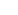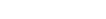How Cheenta works to ensure student success?
Explore the Back-Story

# GCD and Primes | PRMO 2017 | Question 29Try this beautiful problem from the PRMO, 2017 based on GCD and Primes.

## GCD and primes - PRMO 2017

For each positive integer n, consider the highest common factorof the two numbers n!+1 and (n+1)! for n<100, find the largest value of.

• is 107
• is 97
• is 840
• cannot be determined from the given information

### Key Concepts

GCD

Primes

Inequalities

PRMO, 2017, Question 29

Elementary Number Theory by David Burton

## Try with Hints

n! +1 is not divisible by 1,2,.....,n (n+1)! divisible by 1,2,....,n thenand (n+1)! not divisible by n+2, n+3,...... then hcf= (n+1)

let n=99, 99! +1 and (100)! hcf=100 not possible as 100 |99! and 100 is non prime

let n=97 96! + 1 and 97! both divisible by 97 then hcf=97.

## Subscribe to Cheenta at Youtube

Try this beautiful problem from the PRMO, 2017 based on GCD and Primes.

## GCD and primes - PRMO 2017

For each positive integer n, consider the highest common factorof the two numbers n!+1 and (n+1)! for n<100, find the largest value of.

• is 107
• is 97
• is 840
• cannot be determined from the given information

### Key Concepts

GCD

Primes

Inequalities

PRMO, 2017, Question 29

Elementary Number Theory by David Burton

## Try with Hints

n! +1 is not divisible by 1,2,.....,n (n+1)! divisible by 1,2,....,n thenand (n+1)! not divisible by n+2, n+3,...... then hcf= (n+1)

let n=99, 99! +1 and (100)! hcf=100 not possible as 100 |99! and 100 is non prime

let n=97 96! + 1 and 97! both divisible by 97 then hcf=97.

## Subscribe to Cheenta at Youtube

This site uses Akismet to reduce spam. Learn how your comment data is processed.

### Knowledge Partner